## Calculator Keyboard - Faster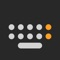# Calculator Keyboard - Faster

by 万庄 黄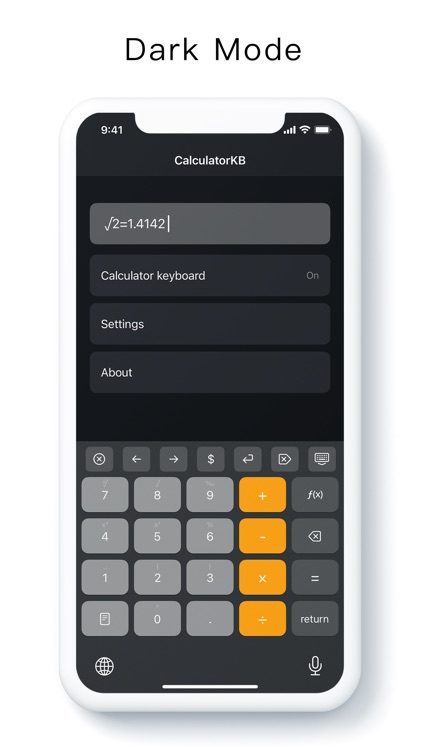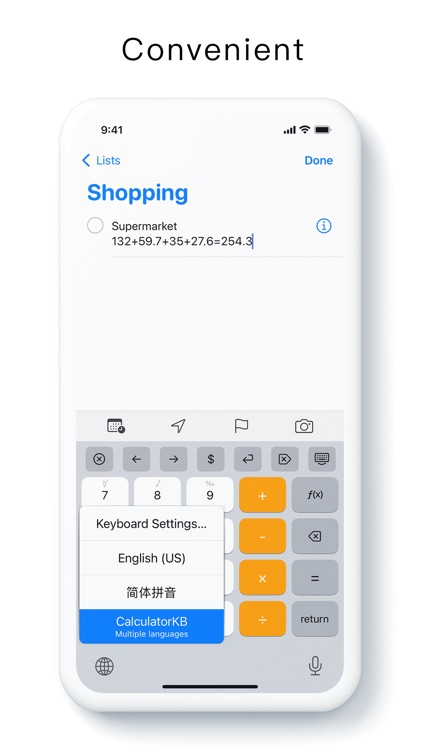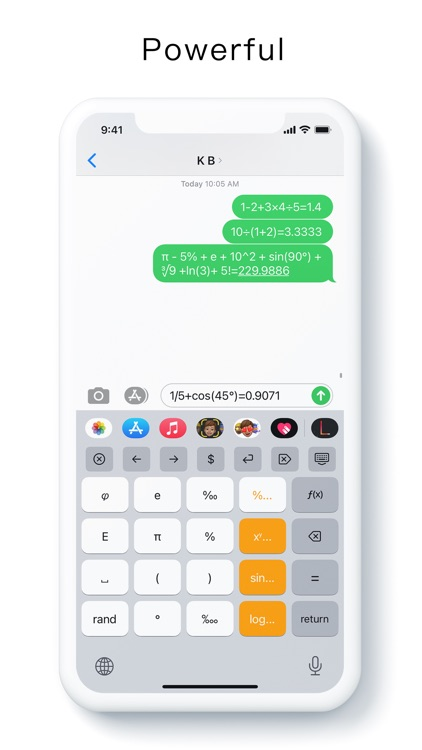Calculator Keyboard - Faster### App Details

Version
1.3.17
Rating
(7)
Size
9Mb
Genre
Productivity Utilities
Last updated
April 26, 2021
Release date
May 19, 2020

### App Screenshots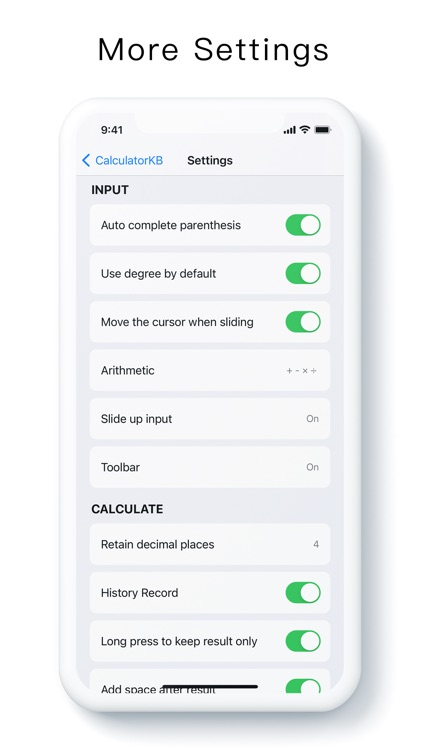### App Store Description

Calculator Keyboard - Faster

Use the "calculator keyboard" to retain the calculation process and quickly calculate the results.
No need to switch between applications, no need to copy and paste, calculate anytime, anywhere, convenient and fast.

[Small] Less than 10 trillion
[Quick] Quick start, fast input, fast calculation

Arithmetic: 1-2 + 3 × 4 ÷ 5 = 1.4

Parentheses: 10 ÷ (1 + 2) = 3.3333

More: π-5% + e + 10 ^ 2 + sin (90 °) + ∛9 + ln (3) + 5! = 229.8815

1/5 + cos (45 °) = 0.9071

10 ÷ 4 = 2.5

Supported operations and functions:

- Arithmetic
- Brackets
- Pi π, percentage%, golden ratio ϕ, natural constant e
- Square x², cubic x³, y power x ^ y
- Square root √, cubic root ∛
- Trigonometric functions sin, cos, tan
- Inverse trigonometric functions asin, acos, atan
- Hyperbolic functions sinh, cosh, tanh
- Natural logarithm ln, constant logarithm log
- Random number rand
- e to the power of x e ^ x
- Countdown 1 / x
- Power of 10 x 10 ^ x

other:

- Custom slide up input
- Custom toolbar
- History record
- Button sound effects
- Key vibration
- Cursor movement
- Implicit multiplication
- Retain decimal places
- Keep results only
- Long press to delete continuously
- Quick input by sliding up
- Automatically complete brackets
- Dark mode

contact: